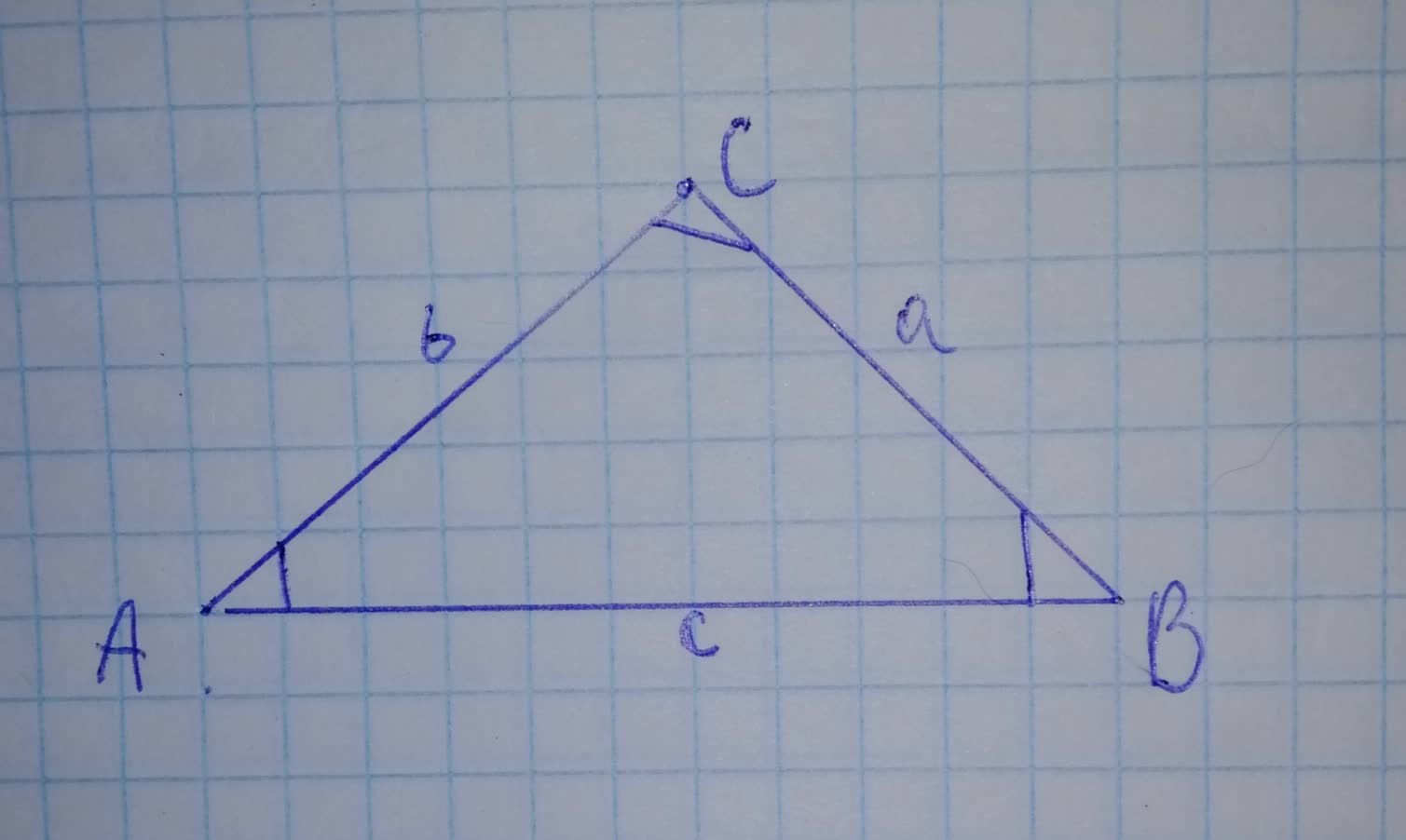# In triangle ABC with sides a, b, and c the Law of Sines states that.xnlghtmarexgo 2021-11-17 Answered
In triangle ABC with sides a, b, and c the Law of Sines states that.
You can still ask an expert for help

• Questions are typically answered in as fast as 30 minutes

Solve your problem for the price of one coffee

• Math expert for every subject
• Pay only if we can solve itFlorence Evans
Step 1
To state the law of sines.
Given information:
A triangle ABC with sides a, b, and c.
Calculation:
It is the relationship between sides and angles of non-right triangles.
Law of sines state that the ratio of length of the side of the non-right-angled triangle of the sine of the opposite angle of that side.
This is same for all angles and sides in an oblique triangle.
In a triangle ABC, the sides are a, b, and c, then
$\frac{a}{\mathrm{sin}\left(A\right)}=\frac{b}{\mathrm{sin}\left(B\right)}=\frac{c}{\mathrm{sin}\left(C\right)}$